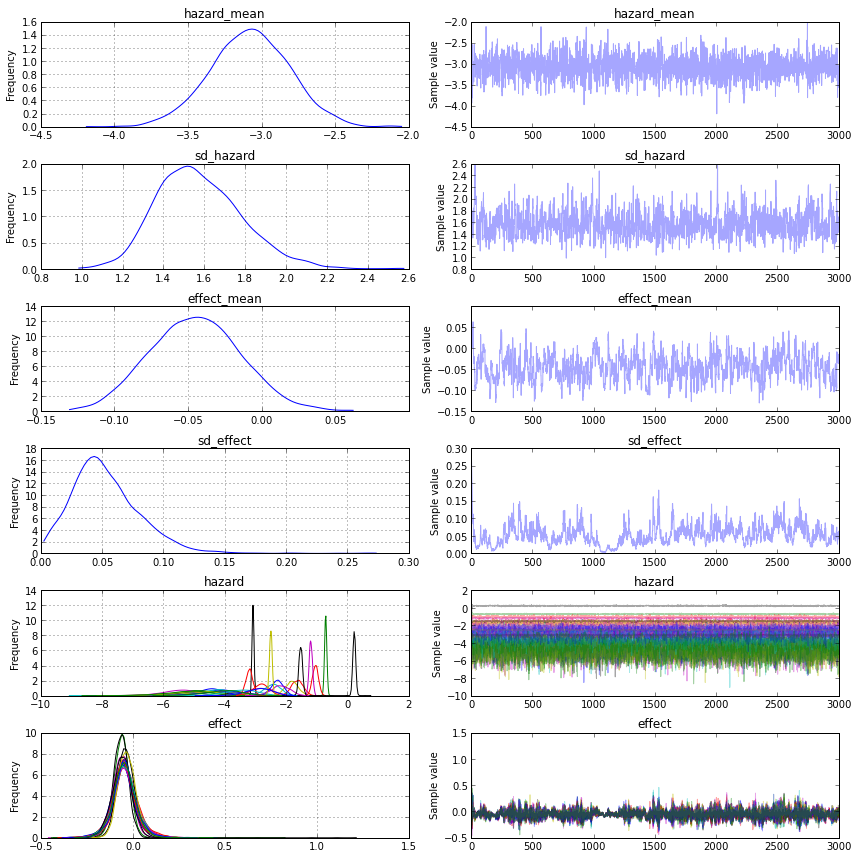## Re-Meta-Analysis of Vitamin D effect on all cause mortality¶

Using Cochrane's meta analysis data.

Bjelakovic G, Gluud LL, Nikolova D, Whitfield K, Wetterslev J, Simonetti RG, Bjelakovic M, Gluud C. Vitamin D supplementation for prevention of mortality in adults. Cochrane Database of Systematic Reviews 2014, Issue 1. Art. No.: CD007470. DOI: 10.1002/14651858.CD007470.pub3.

In :
from lxml import etree
from lxml import objectify

In :
cr = etree.parse("CD007470StatsDataOnly.rm5")
#pulls out all the studies with low risk of bias
root = cr.getroot()
studies_xml = a.getchildren()[1:]#+ a.getchildren()[1:]

def toNumber(v):
try:
return float(v)
except Exception:
return v

def asNumbers(d):
return { k : toNumber(v) for k,v in d.items()}

stds = [asNumbers(dict(s.attrib)) for s in studies_xml]
import pandas as pd
studies = pd.DataFrame(stds)

In :
from pymc import *

In :
def inv_logit(p):
return 1 /(1+ exp(-p))

with Model() as model:
nstudies = len(studies)

hm = Normal('hazard_mean', -2.5, 3) #mean hazard rate
sdh = Tpos('sd_hazard', 15, 1, .5**-2)

#hyperparameters for effect size distribution
em = T('effect_mean', 8, 0, .05**-2) #mean effect size
sd = Tpos('sd_effect', 15, .05, .05**-2)

h = Normal('hazard', hm, sd = sdh, shape = nstudies)
e = Normal('effect', em, sd = sd, shape = nstudies)

controls = Binomial('controls', p = inv_logit(h), n = studies.TOTAL_2.values, observed = studies.EVENTS_2.values)
treatment = Binomial('treatment', p = inv_logit(h+e), n = studies.TOTAL_1.values, observed = studies.EVENTS_1.values)

start = find_MAP(vars =[hm, em, h, e])
step = NUTS(scaling=start)
trace = sample(3000, step, progressbar=False)

INFO (theano.gof.compilelock): Waiting for existing lock by process '12157' (I am process '12197')
INFO (theano.gof.compilelock): To manually release the lock, delete /home/john/.theano/compiledir_Linux-3.2.0-57-generic-pae-i686-with-Ubuntu-12.04-precise-i686-2.7.3-32/lock_dir
/usr/local/lib/python2.7/dist-packages/theano/scan_module/scan_perform_ext.py:85: RuntimeWarning: numpy.ndarray size changed, may indicate binary incompatibility
from scan_perform.scan_perform import *

In :
traceplot(trace);In :
esamples = pd.DataFrame(trace[em])
print "probability mean effect is negative", (esamples < 0).mean()
print "mean effect estimate", esamples.mean()

probability mean effect is negative 0    0.928333
dtype: float64
mean effect estimate 0   -0.044852
dtype: float64

In [ ]: